TWiki>Main Web>MatiasAlejandroBonaventura>TDAQSimulation>TDAQSimulationGroupCommunication>PhaseIFirstSimulations (2016-07-05, MatiasAlejandroBonaventura)

# Simulators comparison (PD, JMT, JMT-MVA) using a super-simple model

## Summary

We compare Powerdevs, JMT discrete and JMT MVA using model which has a source (FelixServer), a Queue+Server (FelixNIC + Link). This represents an application (FelixServer) that sends data through a channel (Link), so data is buffered in the NIC (FelixNIC).

Conclusion: the 3 simulations give similar results. Standard Deviation in PowerDEVS is bigger than JMT's confidence interval. They differ a bit when close to the saturation.
Using different distributions (other than exponential) modifies results, specially close to system saturation.
Performance: MVA is obviously really fast. It can be seen that JMT performs 5 times better than PowerDEVS.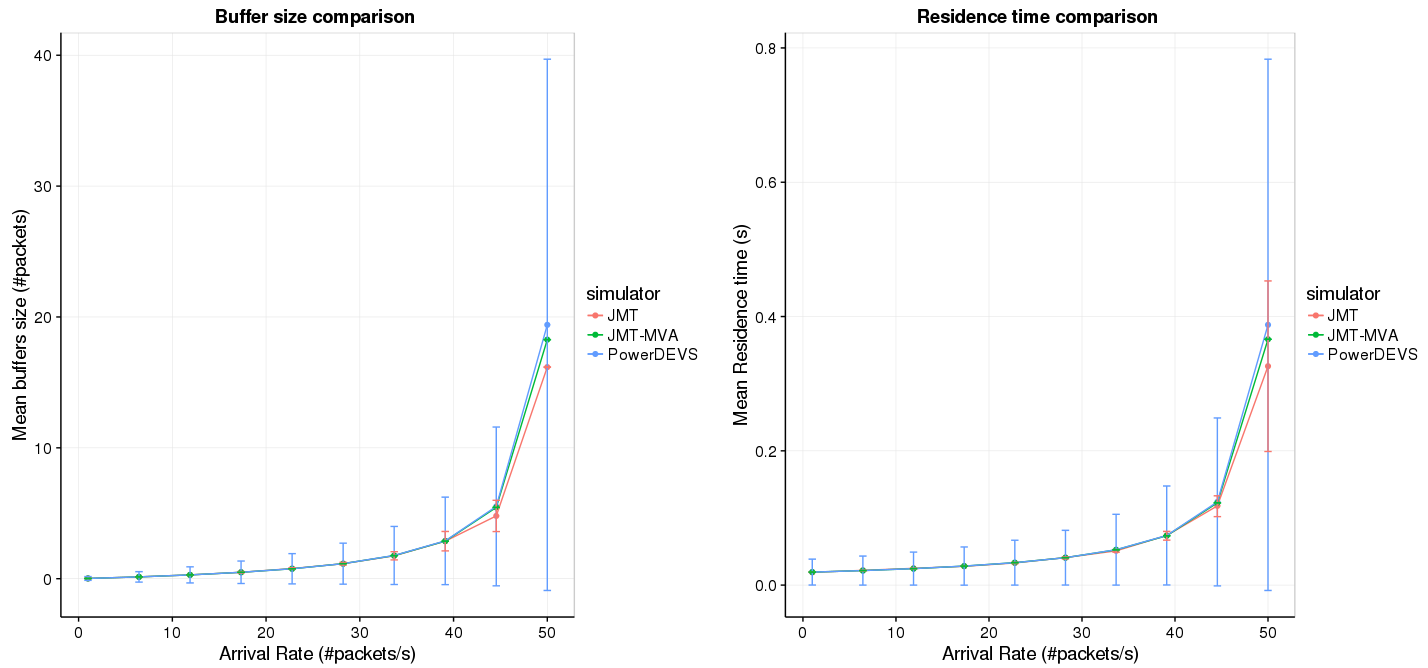NOTE on error bars and means: MVA does not provide confidence interval or sd, so no error bars are plot for MVA
The dots are mean values for the queue size. JMT calculates it with confidence intervals. For powerdevs, we average all measured queue lenghts (this is not a weighted avg as one would naturally think.. see "Averages" for notes on that).

### configuration

rate = swap [1 , 6.444 , 11.889, 17.333, 22.778, 28.222, 33.667, 39.111, 44.556, 50 ]^-1;
FelixServer.period = exp(rate)
FelixServer.packetSize = exp(8000) // packets of size 8000 bits (1 KByte)

Link.Capacity = 421052 // => mean(ServiceTime) = 0.019s

We use an exponential distribution for packetSize (which is unreal) so that it can be studied using MVA (exponential service times required).

We are setting ServiceRate = 0.019 so that the system does not saturate at 50hz (can be studied with MVA), but it is at its limit capacity.

### How we calculate averages - sampler

These tests were performed to validate the Sampler atomic model. The sampler receives events with the QueueSize every time it changes, but logs only every a fixed time period (ej: 1s). When this period elapses the sampler logs the following metrics: max, min, sum, count, timeAvg.

TimeAvg definition
The first 4 metrics are obvious to understand. The 'timeAvg' metric is a time weighted average of the received values. I.E: SUM_1_N( valueN * etN) / samplingPeriod, where value1.. valueN are the received values, and et1 ..et N are the elapsed time where the valueN was observed.
EJ: if the sampling at 1s and receives the following values (t, value): (0.1, 1), (0.5, 5), (0.8, 9), then the metrics would be: max=9. , min=1, count=3, sum=15, timeAvg=(1*0.4 + 5*0.3 + 9*0.2)=3.7

Using the sampler, the "normal" avg can be calculated as sum(sampler_sum) / sum(sampler_count)

It is important to note that the *timeAvg = avg.*
For example the avg in the previous example would be (1+5+9)/3 = 5.

Averages in our model

It is important to note also that the sampler only records one value per timestamp. That means that if it receives 2 values for the same simulated time, it only records the last one.

This is important because the queue publishes queueSize values everytime the queueSize changes, but it can change more than once in the same instant (ej: if the server requested next packet and the queue was empty, when the queue receives next packet it will publish queueSize=1 and in the same instant queueSize=0).

Because of this difference, mean(queue.size) = samplerAvg.
For example, if the server has serviceTime=0 it will instantly request new jobs to the queue. The queue will publish queue.size of 0 and 1: (0,0); (0.1,1); (0.1,0); (0.2, 1); (0.2,0).. etc. Then mean(queue.size)=0.5. The samplerAvg=sum(sampler.sum)/sum(sampler.count) = 0

The samplerAvg seems more accurate of reality, and in practice they don't differ much with reasonable loads.

But in practice there is a big difference between the samplerAvg and the sampler.timeAvg. Although the sampler.timeAvg seems more accurate, it does not match with the MVA averages.
That the reason we use the samplerAvg as metric.

### Models in PD and JMT

Model in PD

Code is commited in GIT. Commit 5eaaa97..2bcec84 (10/6/2016)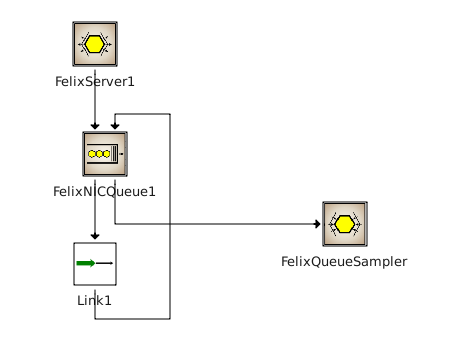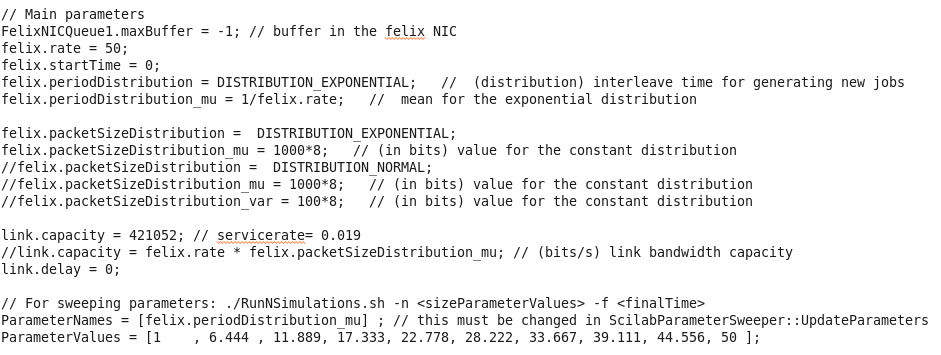model in JMT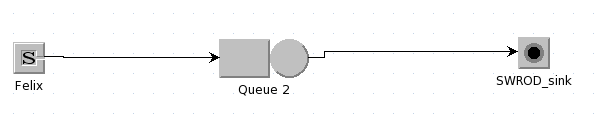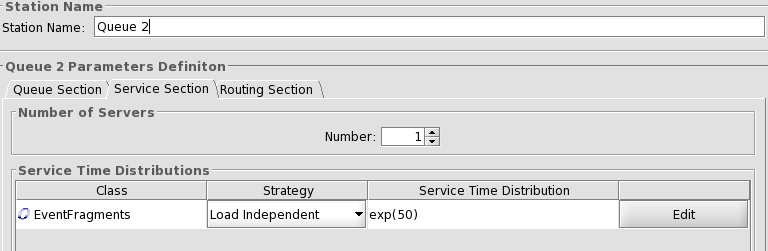### Results

The main results we are looking for in the queueLenght at the FelixNIC size and response time. The what-if scenarios were summarized at the begging, here we show a single run at 50Hz

#### Performance comparison

We compare execution time performance of the different simulators.
It can be seen that JMT performs 5 times better than PowerDEVS.

Configuration:
We simulate 100K seconds at varying arrival rates (from 1 to 50 Hz). So, at the highest rate the simulators produced ~5M new packets.
PowerDEVS can be configured to log more (or less) information, all the simulation with the same sampling rate (set to 1s and 7000s). We sample the queueLenght (7 variables), and at "Full logging" we record ~25 variables.
JMT adjusts the sampling rate dynamically. At 1Hz it recorded 2 samples. At 50Hz it recorded 14 samples. So a fair comparison (sampling wise) would be against PD sampling at 7000s.

Results

MVA has a very low constant time (~100ms), independent of the arrival rate. The other simulators show a linear correlation with the events produced.
JMT has a much softer acceleration respect to the arrival rate (can process ~400K packets in 1 second of execution).
PowerDEVS acceleration depends on the amount of variables logged. In the best case it is 5 times slower than JMT (can process 81K packets in 1 second of execution).

PowerDEVS linear approximation: T(rate) =6.581632653*rate + 9.35.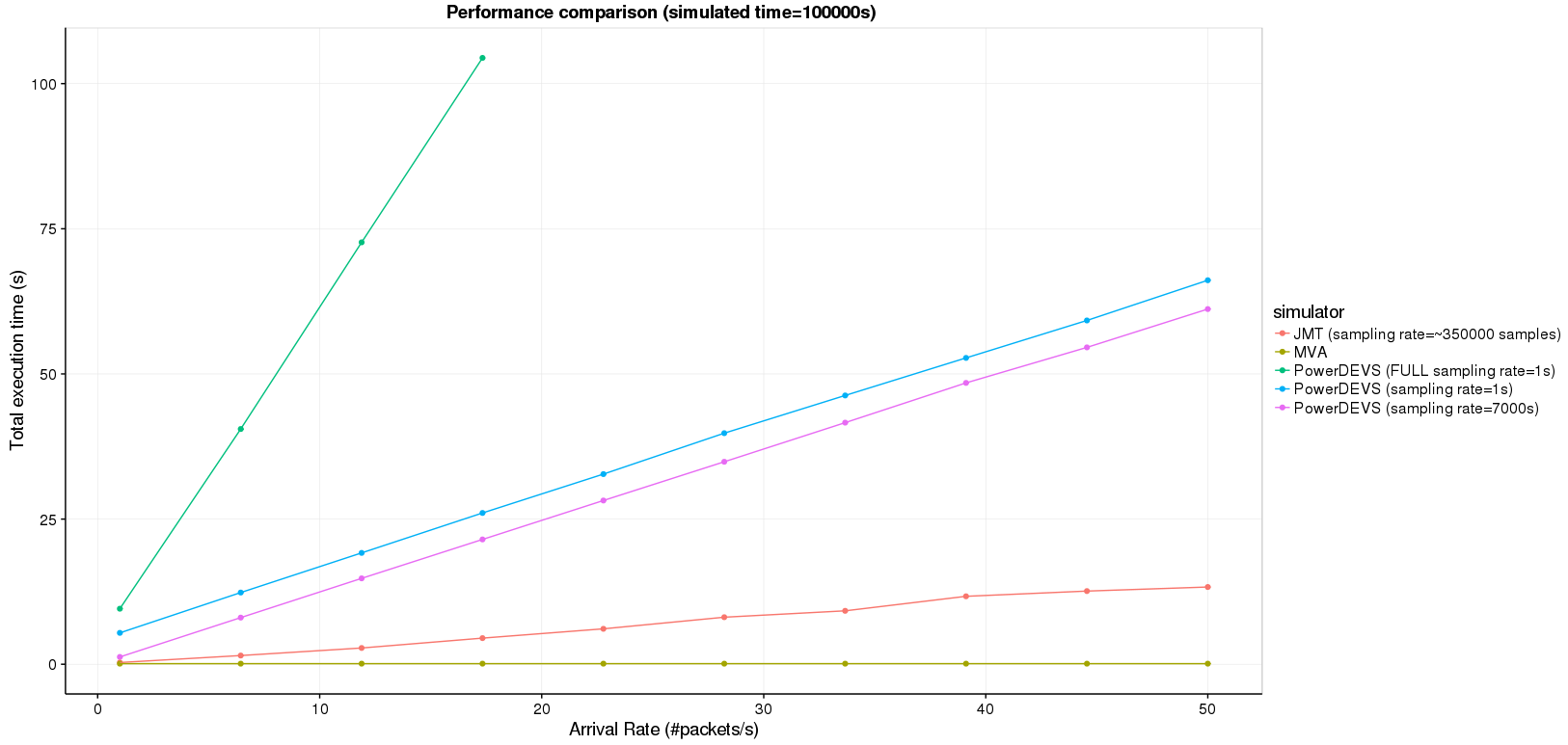#### PowerDEVS - single run

Conditions: We simulate for 1000s. Sampling all values and sampling (both to test sampling differences). Validated distribution and service times with histograms.
Performance: (execution 5.7s with full logging, 0.6 without logging).

Summary: mean queue size = 17.53 packets

Running 20 simulations, each simulation yield a different avg queue size. This is a boxplot for the 20 simulations (the mean is 17.53)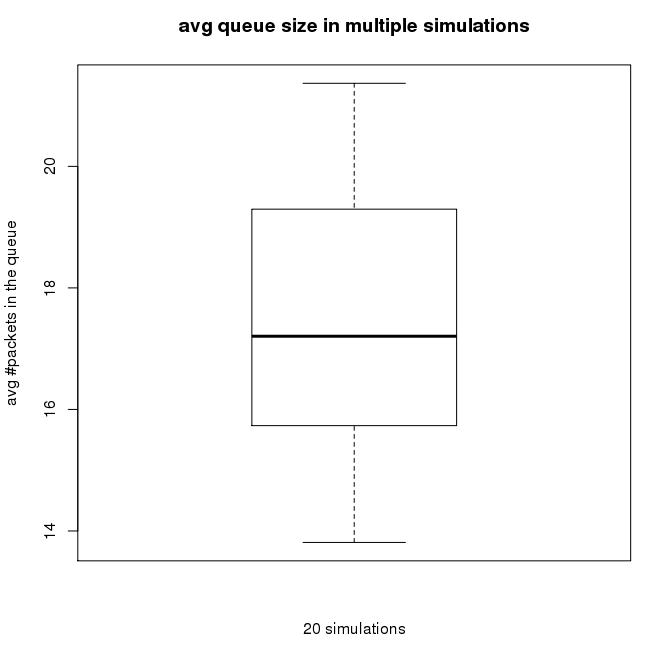#### JMT (Discrete) - single run

Results in JMT discrete:
Conditions: default confidenceInt and error. 2 different simulatios stop rules: 1) with a limit of 9M samples and 2) with a limit of 1000 simualted seconds (like in PD).
#customers shows that confidenceInt was not met.

Summary: mean queue size = 20.9 packets
Permormance: 30.2s for 9M samples, 0.4s to simulate 1000s.
#OfCustomers.avg = 19.33
ResidenceTime = 0.39

1000s simualted
0.4s of execution --> SUPER FAST!!!
93M samples --> strange because it was configured to generate 50Hz so in 1000s~50M samples (I run it several times and simular amount of samples)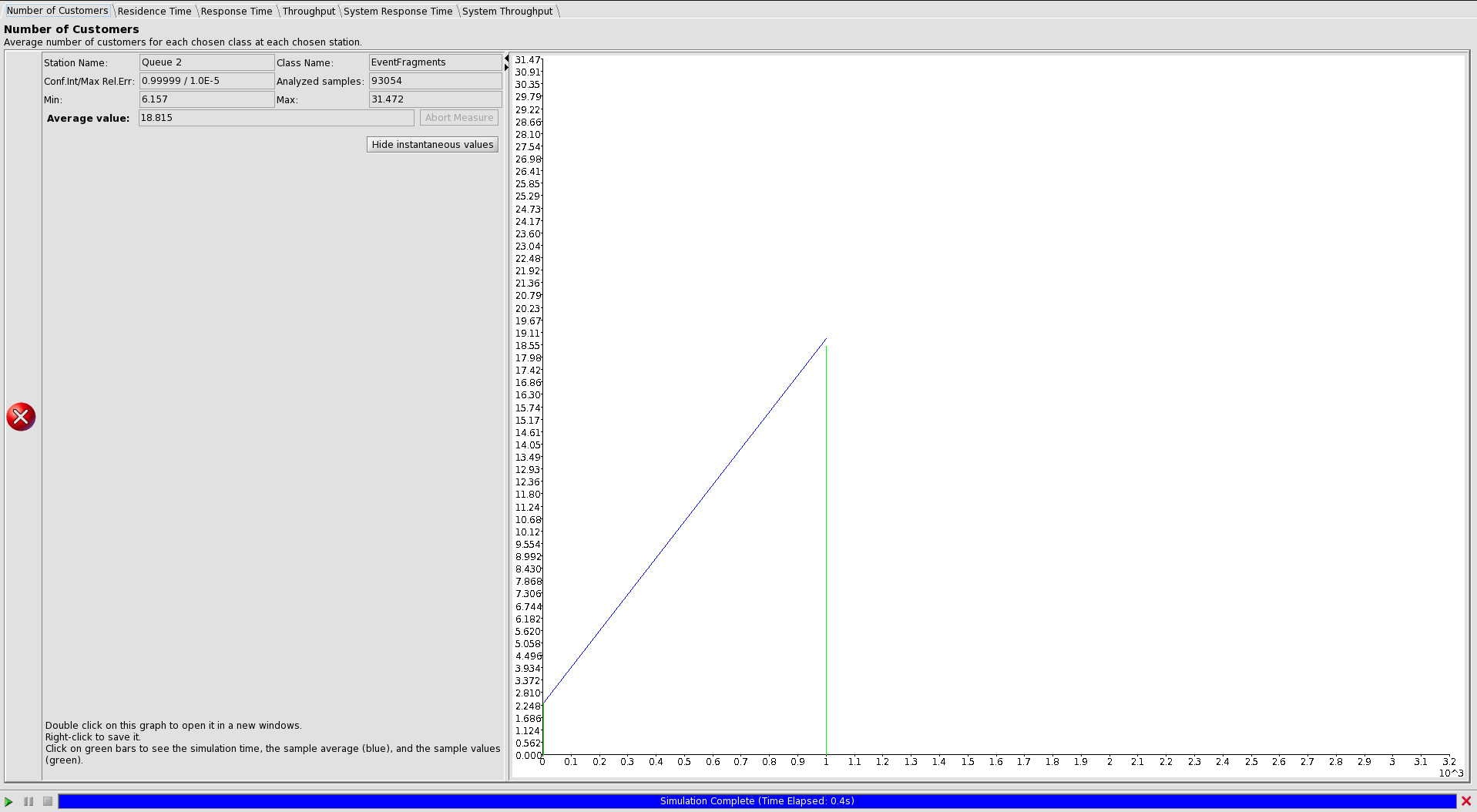9M samples
29.2s execution --> FAST!
180M simulated seconds --> this does match with the #samples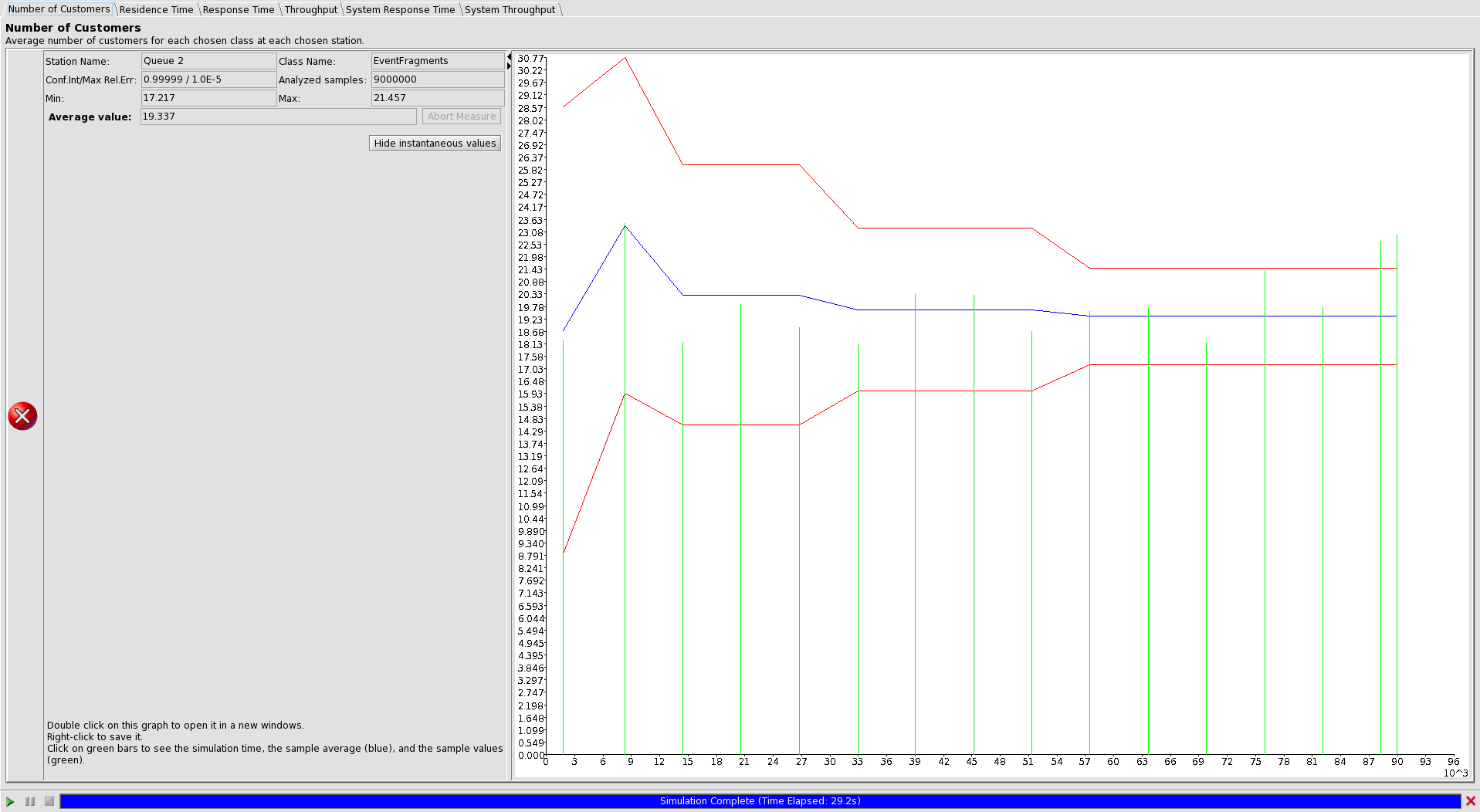#### JMT - MVA - single run

NumberOfCustomers.avg = 19
ResidenceTime = 0.38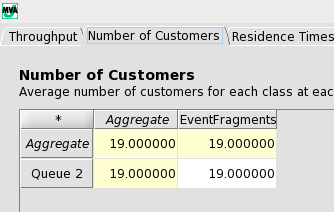## Comparison using different Distributions (other than exponential)

Here we compare results when an exponential distribution is used with other distributions (NORMAL, CONSTANT, etc)

why? rationale
MVA can only be used when the arrivalRate and serviceTime follow exponential distributions. The arrivalRate in networks can have bursts. The serviceTime depends on the packetSizes, which they might follow a Normal distribution.

### ServiceTime (packetSize)

The plot compares the results using different distributions for the packetSize. The exp distribution is left as reference, which would yield similar results to MVA.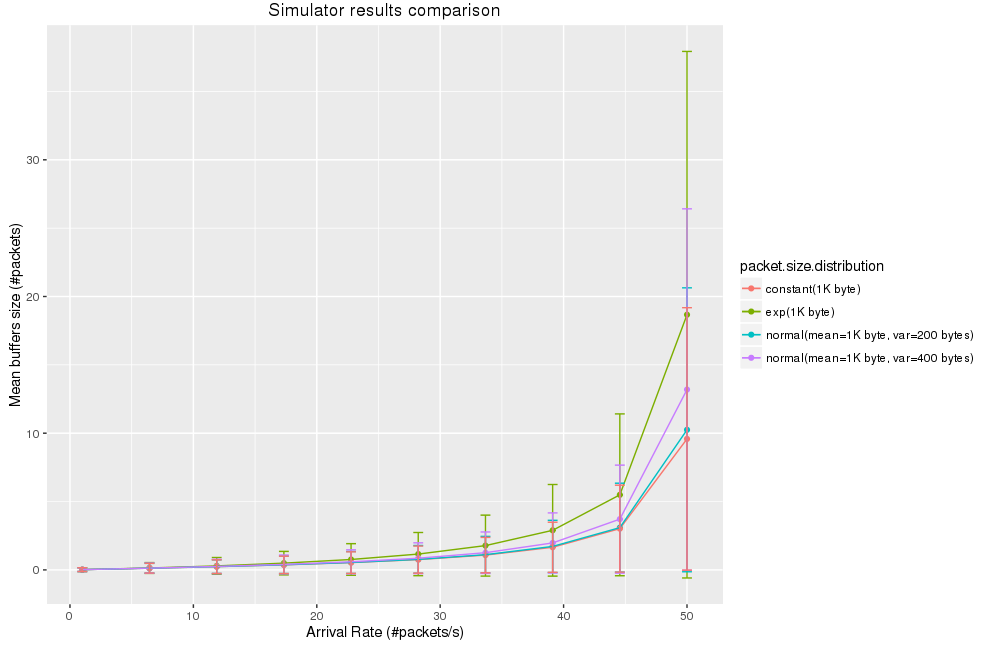-- MatiasAlejandroBonaventura - 2016-06-06

mean(FelixQueueSampler _timeAv)

Topic revision: r11 - 2016-07-05 - MatiasAlejandroBonaventuraLog In

Webs

Welcome GuestCern SearchTWiki SearchGoogle Search Main All websCopyright &© 2008-2020 by the contributing authors. All material on this collaboration platform is the property of the contributing authors.
Ideas, requests, problems regarding TWiki? Send feedback